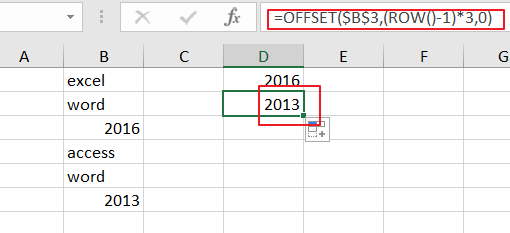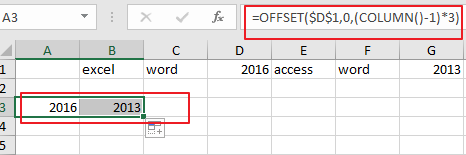# How To Increment Cell Reference by X Rows in Excel

This post will guide you how to increment cell reference by x Rows when you drag a formula to populate cells in a column in Excel. By default, when you drag the AutoFill Handle down to other cells , the cell reference in the formulas will be increated by one. And if you want to increase cell reference by a given number，how to do it.

Assuming that you have a list of data in range B1:B6, and you have a formula in another cell, and you want to increase cell references by 3 rows when dragging AutoFill handle. type：

`=OFFSET(\$B\$3,(ROW()-1)*3,0)`

Note: Cell \$B\$3 is the first cell tha tyou want to extract in your data.

Dragging this formula down to other cells in that column, you would see that the cell references are increased by 3 rows.If you want to increase cell reference by 3 columns in a row range, just using the following formula:

`=OFFSET(\$D\$1,0,(COLUMN()-1)*3)`

Type this formula into the first cell in a new row, press Enter key ,and drag the AutoFill Handle to other cells.### Related Functions

• Excel ROW function
The Excel ROW function returns the row number of a cell reference.The ROW function is a build-in function in Microsoft Excel and it is categorized as a Lookup and Reference Function.The syntax of the ROW function is as below:= ROW ([reference])….
• Excel COLUMN function
The Excel COLUMN function returns the first column number of the given cell reference.The syntax of the COLUMN function is as below:=COLUMN ([reference])….